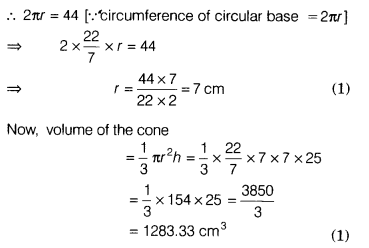# If the circumference of the base of a cone 44cm and its height 25cm, then find the volume of cone

If the circumference of the base of a cone is 44 cm and its height is 25 cm, then find the volume of the cone.

Let r be the radius of the base of a cone.
Given, height, h = 25 cm
and circumference of the base of a cone = 44 cm